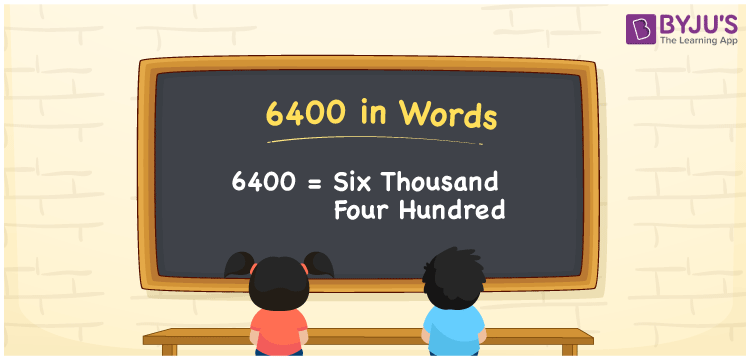# 6400 in Words

6400 in words is written as “Six Thousand Four Hundred”. In Maths, 6400 is a cardinal number that expresses a quantity. To write a cheque for Rs.6400, we can write in words as Rupees Six Thousand Four Hundred only. Also, using it as a counting number, we can say, there are 6400 people in a cricket stadium. Learn more about Numbers In Words and writing the number names in English at BYJU’S.

 6400 in Words Six Thousand Four Hundred Six Thousand Four Hundred in Numbers 6400

## 6400 in English Words

To make it easy for children to learn the names of the numbers we have represented the chart below. It shows the name of 6400 in English words.## How to Write 6400 in Words?

The number 6400 in words can be written using a place value system, where we can identify the position of each digit of the original number. Since 6400 is a four-digit number, thus,

 Thousands Hundreds Tens Ones 6 4 0 0

From the above table,

6 → Thousands

4 → Hundreds

0 → Tens

0 → Ones

Hence, when we read the number from right to left, it is given by Six Thousand Four Hundred.

### Expanded Form of 6400

We can write the expanded form as:

6 x Thousand + 4 × Hundred + 0 × Ten + 0 × One

= 6 x 1000 + 4 × 100 + 0 × 10 + 0 × 1

= 6000 + 400 + 0 + 0

= Six Thousand Four Hundred

6400 is a whole number that is succeeded by 6399 and preceded by 6401. Learn more about the number 6400 below:

• 6400 in Words – Six Thousand Four Hundred
• Is 6400 an odd number? – No
• Is 6400 an even number? – Yes
• Is 6400 a perfect square number? – Yes (√6400 = 80)
• Is 6400 a perfect cube number? – No
• Is 6400 a prime number? – No
• Is 6400 a composite number? – Yes

## Frequently Asked Questions on 6400 in words

Q1

### What is 6400 in words?

6400 in words is given by Six Thousand Four Hundred.
Q2

### What is the rule to write 6400 in words?

To write the 6400 in words, we should use place value rule, where the position of each digit helps in determining the name of the number. In 6400, 6 is at Thousands, 4 is at Hundreds, 0 is at Tens and Ones place. Hence, the number is read as Six Thousand Four Hundred.
Q3

### What is the value of 6400 + 600 in words?

6400 + 600 = 7000, i.e., Seven Thousand in words.